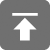# 工学1号馆

home

## C面向对象编程–抽象数据类型

Wu Yudong    September 11, 2016     C   510

## 一、数据类型：

typedef  struct node {
struct node *next;
//...information...
}  node;

node * head(node * elt, const node * tail);

## 三、例子–Set

#ifndef    SET_H
#define    SET_H
extern const void * Set;
void * add (void * set, const void * element);
void * find (const void * set, const void * element);
void * drop (void * set, const void * element);
int contains (const void * set, const void * element);
unsigned count (const void * set);
#endif

Set将在某种程度上展示我们在sets上的操作，add( )向set中添加一个元素，返回是否已经存在于set或是添加成功，find( )在set中寻找一个元素，返回位置或是空指针。drop( )定位一个元素并从set中移除，返回移除元素。contains( )将find( )的结果转换为一个具体的值。

## 四、内存管理

#ifndef    NEW_H
#define    NEW_H
void * new (const void * type, ...);
void delete (void * item);
#endif

## 五、Object

#ifndef    OBJECT_H
#define    OBJECT_H
extern const void * Object;        /* new(Object); */
int differ (const void * a, const void * b);
#endif

## 六、应用

#include <stdio.h>

#include "new.h"
#include "Object.h"
#include "Set.h"

int main ()
{    void * s = new(Set);
void * a = add(s, new(Object));
void * b = add(s, new(Object));
void * c = new(Object);

if (contains(s, a) && contains(s, b))
puts("ok");

if (contains(s, c))
puts("contains?");

puts("differ?");

if (contains(s, drop(s, a)))
puts("drop?");

delete(drop(s, b));
delete(drop(s, c));

return 0;
}

## 七、实现

main.c 可以成功编译，但是在编译和执行程序之前，我们必须实现抽象数据类型和内存管理，如果一个对象不储存任何信息，并且每一个对象都至少属于一个set，那么我们可以用一个唯一的较小的正整数值来表示对象和每一个set，而这些正整数值可以使用一个数组heap[ ]中的索引来表示。

Sets和对象具有相同的展示，new( )不会在意type的类型描述，它将返回heap[ ]中值为0的元素，代码如下:

#if ! defined MANY || MANY < 1
#define    MANY    10
#endif

static int heap [MANY];

void * new (const void * type, ...)
{    int * p;                            /* & heap[1..] */

for (p = heap + 1; p < heap + MANY; ++ p)
if (! * p)
break;
assert(p < heap + MANY);
* p = MANY;
return p;
}

void delete (void * _item)
{    int * item = _item;

if (item)
{    assert(item > heap && item < heap + MANY);
* item = 0;
}
}

void * add (void * _set, const void * _element)
{    int * set = _set;
const int * element = _element;

assert(set > heap && set < heap + MANY);
assert(* set == MANY);
assert(element > heap && element < heap + MANY);

if (* element == MANY)
* (int *) element = set - heap;
else
assert(* element == set - heap);

return (void *) element;
}

void * find (const void * _set, const void * _element)
{   const int * set = _set;
const int * element = _element;

assert(set > heap && set < heap + MANY);
assert(* set == MANY);
assert(element > heap && element < heap + MANY);
assert(* element);

return * element == set - heap ? (void *) element : 0;
}

contains( )将find( ) 得到的结果转换为一个真值：

int contains (const void * _set, const void * _element)
{
return find(_set, _element) != 0;
}

drop( ) 依赖find( )函数来检查要删除的元素是否在set中，若是，则通过将相应的对象元素的值标记为MANY：

void * drop (void * _set, const void * _element)
{    int * element = find(_set, _element);

if (element)
* element = MANY;
return element;
}

int differ (const void * a, const void * b)
{
return a != b;
}

#include <assert.h>
#include <stdio.h>

#include "new.h"
#include "Set.h"
#include "Object.h"

const void * Set;
const void * Object;

#if ! defined MANY || MANY < 1
#define    MANY    10
#endif

static int heap [MANY];

void * new (const void * type, ...)
{    int * p;                            /* & heap[1..] */

for (p = heap + 1; p < heap + MANY; ++ p)
if (! * p)
break;
assert(p < heap + MANY);
* p = MANY;
return p;
}

void delete (void * _item)
{    int * item = _item;

if (item)
{    assert(item > heap && item < heap + MANY);
* item = 0;
}
}

void * add (void * _set, const void * _element)
{    int * set = _set;
const int * element = _element;

assert(set > heap && set < heap + MANY);
assert(* set == MANY);
assert(element > heap && element < heap + MANY);

if (* element == MANY)
* (int *) element = set - heap;
else
assert(* element == set - heap);

return (void *) element;
}

void * find (const void * _set, const void * _element)
{    const int * set = _set;
const int * element = _element;

assert(set > heap && set < heap + MANY);
assert(* set == MANY);
assert(element > heap && element < heap + MANY);
assert(* element);

return * element == set - heap ? (void *) element : 0;
}

int contains (const void * _set, const void * _element)
{
return find(_set, _element) != 0;
}

void * drop (void * _set, const void * _element)
{    int * element = find(_set, _element);

if (element)
* element = MANY;
return element;
}

int differ (const void * a, const void * b)
{
return a != b;
}